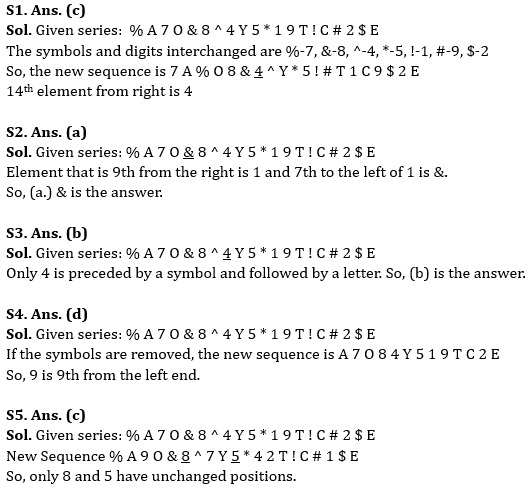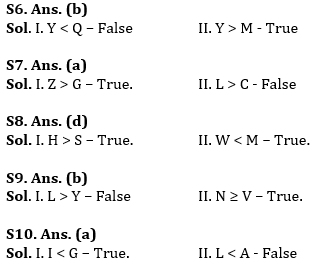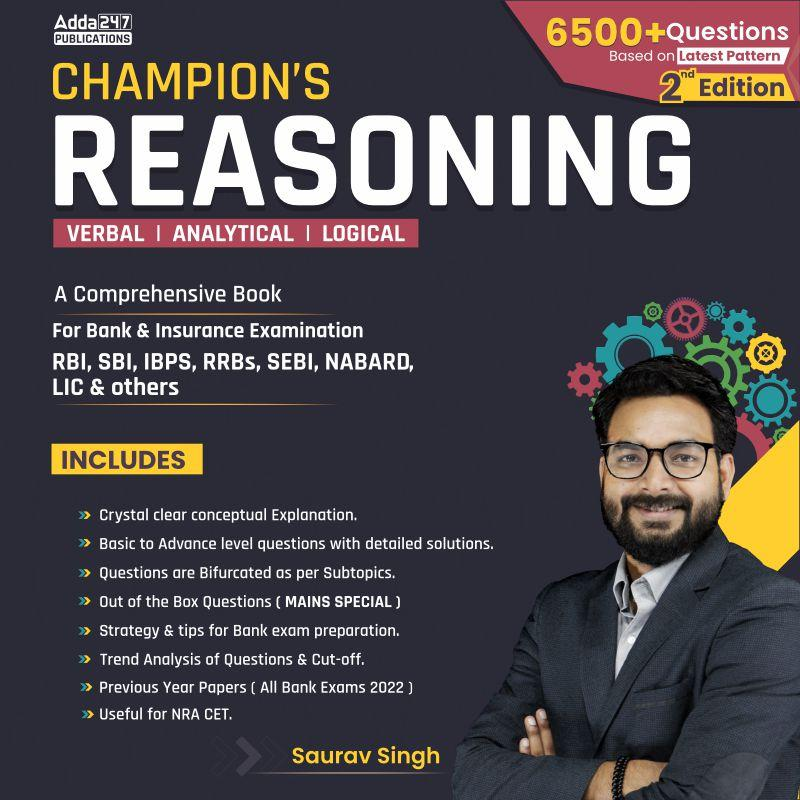Latest Banking jobs   »   IBPS reasoning

# Reasoning Quiz For IBPS PO Prelims 2023-18th September

Directions (1-5): Answer the following questions based on the alphanumeric symbol series given below:
% A 7 O & 8 ^ 4 Y 5 * 1 9 T ! C # 2 \$ E

Q1. If the digits and symbols are interchanged i.e., the first digit is interchanged with the first symbol, second digit with the second symbol and so on from the left end, which will be the 14th element from the right end?
(a) ^
(b) 8
(c) 4
(d) &
(e) T

Q2. Which is the 7th element to the left of the element which is 9th from the right end in the series?
(a) &
(b) C
(c) 2
(d) #
(e) \$

Q3. How many digits are there in the series which are immediately preceded by a symbol and immediately followed by a letter?
(a) 0
(b) 1
(c) 2
(d) 3
(e) More than 3

Q4. If all the symbols are removed from the series, then which will be the element which is 9th from the left end?
(a) 4
(b) 7
(c) 1
(d) 9
(e) None of the above

Q5. If all the digits are arranged in decreasing order from the left, how many digits will have their positions unchanged?
(a) 0
(b) 1
(c) 2
(d) 3
(e) More than 3

Directions (6-10): In the following questions assuming the given statement to be true, then find which of the following conclusion(s) among given conclusions is/are definitely true and then give your answers accordingly.

Q6. Statements: Q > A = E ≥ S = T, J = A > L ≥ M, Y = R ≥ A > O
Conclusions: I. Y < Q
II. Y > M
(a) Only conclusion I is true
(b) Only conclusion II is true
(c) Either conclusion I or II is true
(d) Both conclusions I and II are true
(e) Neither conclusion I nor II is true

Q7. Statements: G < Q = C ≤ N = X, S > N = E > M, Z = J = S > L
Conclusions: I. Z > G
II. L > C
(a) Only conclusion I is true
(b) Only conclusion II is true
(c) Either conclusion I or II is true
(d) Both conclusions I and II are true
(e) Neither conclusion I nor II is true

Q8. Statements: N ≤ T = H < M, N = W ≥ K > S, P ≤ M = D
Conclusions: I. H > S
II. W < M
(a) Only conclusion I is true
(b) Only conclusion II is true
(c) Either conclusion I or II is true
(d) Both conclusions I and II are true
(e) Neither conclusion I nor II is true

Q9. Statements: V ≤ P = C ≤ H, N ≥ C < E = Y, P = L ≤ S = T
Conclusions: I. L > Y
II. N ≥ V
(a) Only conclusion I is true
(b) Only conclusion II is true
(c) Either conclusion I or II is true
(d) Both conclusions I and II are true
(e) Neither conclusion I nor II is true

Q10. Statements: I = E > W < L, D ≤ F = E < X, X < T = G > A
Conclusions: I. I < G
II. L < A
(a) Only conclusion I is true
(b) Only conclusion II is true
(c) Either conclusion I or II is true
(d) Both conclusions I and II are true
(e) Neither conclusion I nor II is true

Solutions## FAQs

### When will the IBPS PO prelims 2023 be conducted?

IBPS PO Prelims will be conducted on 23, 30 September, and 1 October 2023.

#### Congratulations!Union Budget 2023-24: Free PDF